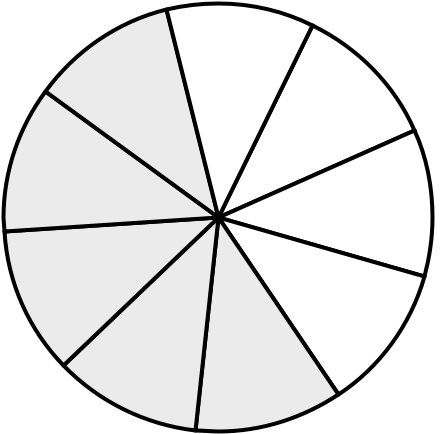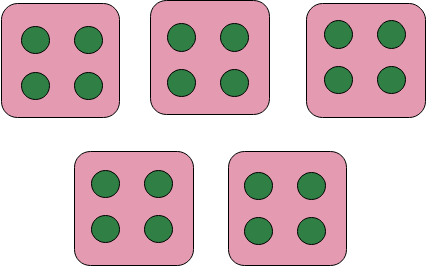Home » Maths » Denominator and Divisor

# Denominator and Divisor

In the study of mathematics, particularly in fractions and division operations, two terms that often arise are denominator and divisor. Let’s explore both concepts in detail.

## Denominator and Divisor

### What is Denominator?

Definition: In a fraction, the denominator represents the total number of equal parts into which a quantity is divided. It is the number below the line in a fractional expression.

Example of Denominator

In the fraction $\frac{5}{8}$, the number 8 is the denominator. This means that the whole is divided into 8 equal parts, and the fraction represents 5 of those parts.The diagram given below illustrates this. It shows a circle and divides it into 8 equal parts. Five shaded parts represent the fraction $\frac{5}{8}$.

Questions:

1. What is the denominator in the fraction $\frac{3}{4}$?
2. How does the value of the denominator affect the value of the fraction?

1. In the fraction $\frac{3}{4}$, the denominator is 4.
2. The larger the denominator, the smaller each part of the whole, and therefore the smaller the value of the fraction (assuming the numerator remains constant).

### 2. Divisor

Definition: In a division operation, the divisor is the number by which another number (called the dividend) is divided.

#### Example of Divisor

In the division operation $\frac{20}{4} = 5$, the number 4 is the divisor. It represents how many times the dividend (20) is to be divided.The diagram above shows an example of a divisor by drawing 20 dots and dividing them into groups of four. There will be five groups to demonstrate that 20 divided by four equals five.

Questions:

1. What is the divisor in the division operation $\frac{15}{3} = 5$?
2. What happens to the quotient if the divisor is increased?

1. In the division operation $\frac{15}{3} = 5$, the divisor is 3.
2. If the divisor is increased, the quotient (result of division) decreases, provided the dividend remains the same.

## Comparison Between Denominator and Divisor

While the denominator and divisor are both related to division, they serve different roles. The denominator tells us into how many equal parts a whole is divided in the context of fractions, while the divisor tells us by what number we are dividing another number in the context of a division operation.

The difference between the denominator and the divisor can be clearly illustrated in the form of a table.

Attribute Denominator Divisor
Definition Number of equal parts in a fraction. Number by which another number is divided.
Context Used in a fraction (e.g. $\frac{a}{b}$). Used in a division operation (e.g. $\frac{a}{b} = c$).
Position Found below the line in a fraction. Used as the ‘dividing by’ number in a division expression.
Effect on Value The larger the denominator, the smaller the value of the fraction (for a fixed numerator). The larger the divisor, the smaller the quotient (for a fixed dividend).
Example In $\frac{5}{8}$, 8 is the denominator. In $\frac{20}{4} = 5$, 4 is the divisor.
Diagram Can be represented by dividing a shape into equal parts. Can be represented by grouping objects into equal sets.

Questions for Reflection:

1. How is the role of the denominator in a fraction different from the role of the divisor in a division operation?
2. Can you think of a mathematical expression where both the concepts of the denominator and divisor are present?

2. In the expression $\frac{\frac{15}{3}}{5}$, 3 is the denominator of the fraction and 5 is the divisor in the overall division.